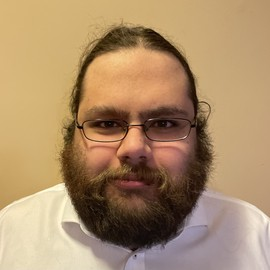## Max Gutkin

### University of Manitoba

I am currently a M.Sc. student at the University of Manitoba studying graph processes on random networks. In the past I have worked on projects involving the burning number of graphs, the chromatic number of great circle graphs, and finding Betti splittings of symbolic powers of edge ideals of complete graphs. My main field of interest is graph theory, with a focus on algorithmic approaches to problems of graph processes.

Interests
• Graph Theory
• Graph Processes
• Algorithm Design and Analysis
• Ideal Theory
Education
• B.Sc. in Computer Science and Mathematics

University of Manitoba